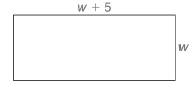Chapter A.4, Problem 37EElementary Geometry For College St...

7th Edition
Alexander + 2 others
ISBN: 9781337614085

Solutions

Chapter
SectionElementary Geometry For College St...

7th Edition
Alexander + 2 others
ISBN: 9781337614085
Textbook Problem

Given that the area of the rectangle shown is 66, find the width w and length w + 5 by solving the equation w w + 5 = 66 .To determine

To find:

The width w and length w+5 by solving the equation ww+5=66.

The area of the rectangle shown below is 66.Explanation

Solving an equation is to find the value of the unknown variables in the equation, such that the obtained value or values of the unknown should satisfy the equation from which it was derived. Such a value is said to be the solution for the equation. In general a quadratic equation has two solutions for the variable in the equation as the degree of the equation is two.

Calculation:

Given,

Length =w+5, and

Width =w.

ww+5=66

Apply the distributive property of multiplication over addition.

w·w+w·5=66

w2+5w=66

Add; -66 on both sides,

w2+5w+-66=66+(-66)

w2+5w+-66=66+(-66)

w2+5w-66=0.

The above equation is in the standard form of a quadratic equation.

Now, we shall proceed with the factorization of the quadratic expression in the left of the equation.

First look for the common factor GCF of the terms w2, 5w and -66.

But, here the GCF is 1.

Thus, we have to factor the above equation by the reverse FOIL method.

First factorize the product of the coefficient of the first tern and the constant, such that the sum of the factors gives the coefficient of the middle term.

Thus, factorize -66 as 11·-6 so that the sum of the factors 11+(-6) gives 5.

Now, split the middle term 5w as 11w+(-6w).

Hence, the equation becomes,

w2+11w+-6w-66=0

w·w+11·w+-6·w+-6·11=0

Factor out the common factors form the first two terms and form the last two terms of the above expression

Still sussing out bartleby?

Check out a sample textbook solution.

See a sample solution

The Solution to Your Study Problems

Bartleby provides explanations to thousands of textbook problems written by our experts, many with advanced degrees!

Get Started

Differentiate the function. y = ln |1 + t t3|

Single Variable Calculus: Early Transcendentals, Volume I

Repeat Exercise 29 for the curve y=x+sinx0x2

Single Variable Calculus: Early Transcendentals

The solution to y = y2 with y(1)=13 is: a) y=1x+2 b) y=lnx+13 c) y=12x216 d) y=12x16

Study Guide for Stewart's Single Variable Calculus: Early Transcendentals, 8th

The distance between (7, 4, –3) and (–1, 2, 3) is: 4 16 104

Study Guide for Stewart's Multivariable Calculus, 8th